微积分奇观之计算曲线的平均高度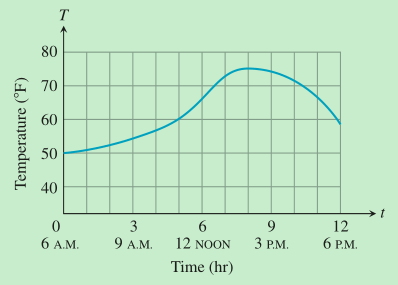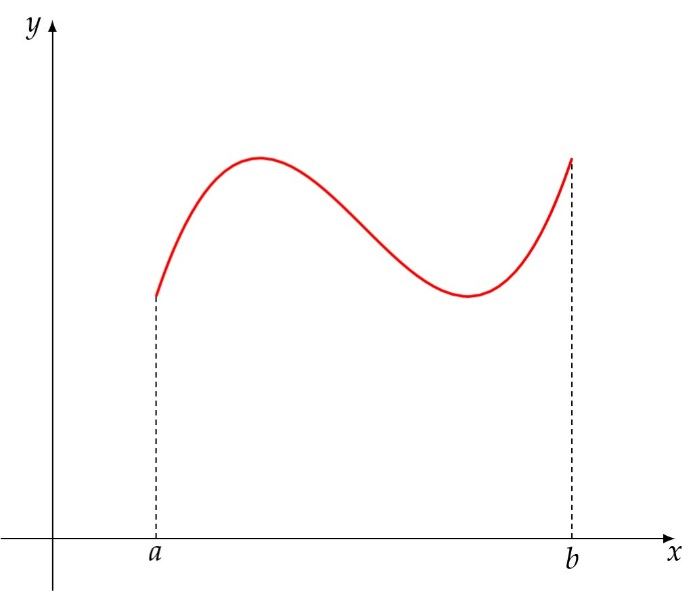a = x0 < x1 < x2 < ⋯ < xi − 1 < xi < ⋯ < xn = b （其中i = 1, 2, …, n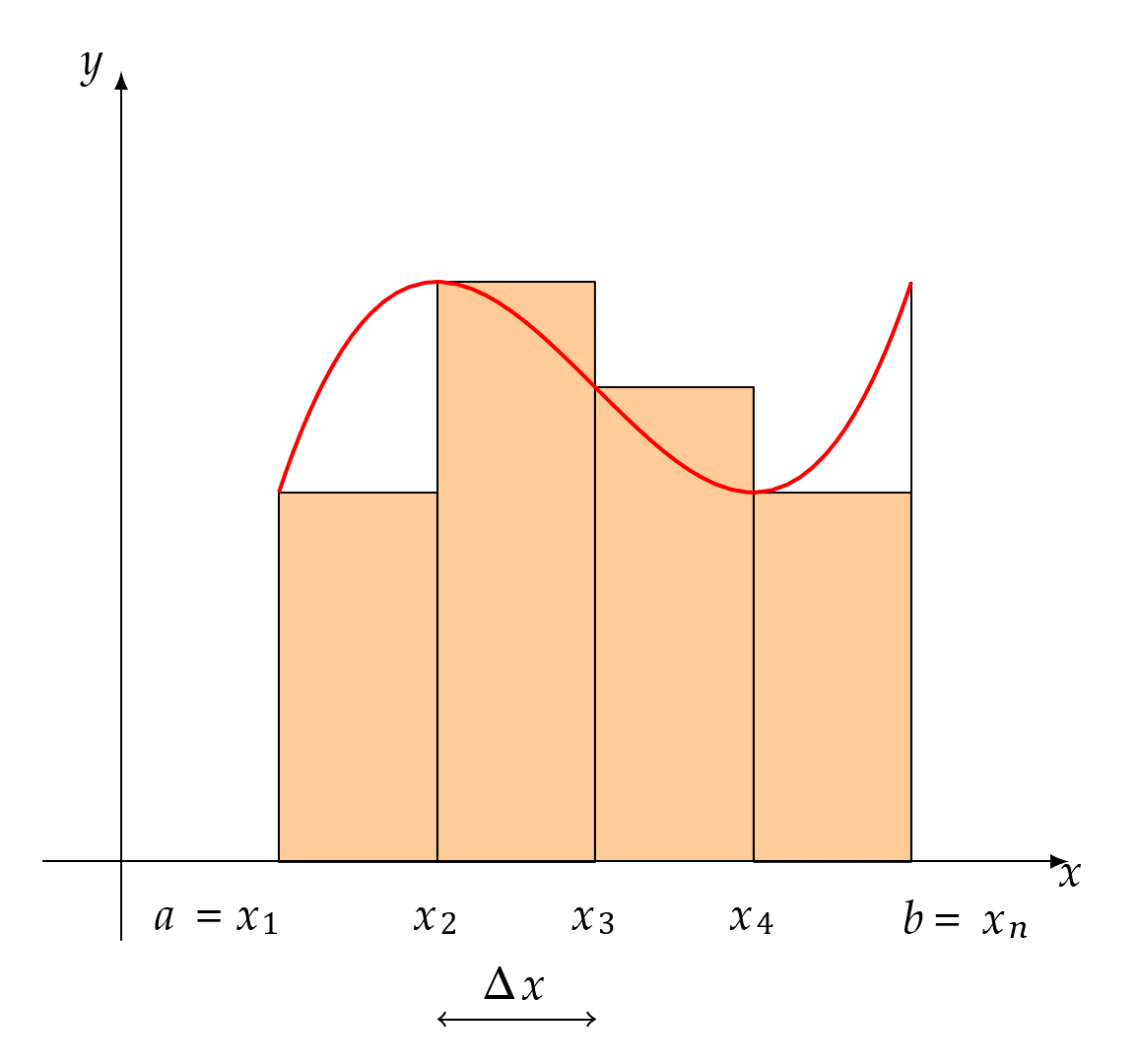1

$$\lim_{n \rightarrow \infty}\frac{f\left( \xi_{1} \right) + f\left( \xi_{2} \right) + \cdots + f\left( \xi_{n} \right)}{n}$$

$$\begin{matrix} \lim_{\lambda \rightarrow 0}\left( f\left( \xi_{1} \right)\text{Δx} + f\left( \xi_{2} \right)\text{Δx} + \cdots + f\left( \xi_{n} \right)\text{Δx} \right) = \lim_{\lambda \rightarrow 0}{\sum_{i = 1}^{n}{f\left( \xi_{i} \right)\text{Δx} = S}} \\ \end{matrix}$$

{\begin{aligned}\lim _{n \rightarrow \infty} \frac{f\left(\xi_{1}\right)+f\left(\xi_{2}\right)+\cdots+f\left(\xi_{n}\right)}{n}&=\lim _{n \rightarrow \infty} \frac{f\left(\xi_{1}\right) \Delta x+f\left(\xi_{2}\right) \Delta x+\cdots+f\left(\xi_{n}\right) \Delta x}{n \Delta x}\\&=\frac{\lim _{n \rightarrow \infty}\left(f\left(\xi_{1}\right) \Delta x+f\left(\xi_{2}\right) \Delta x+\cdots+f\left(\xi_{n}\right) \Delta x\right)}{\lim _{n \rightarrow \infty}(n \Delta x)}\\&=\frac{S}{\lim _{n \rightarrow \infty}\left(n \times \frac{b-a}{n}\right)}\\&=\frac{S}{b-a}\end{aligned}}

S是曲边梯形的面积，而b − a是曲边梯形的底边长度，所以求出来的连续曲线f(x)于[a,b]上所有点的平均高度$\frac{S}{b - a}$就相当于是与曲边梯形面积相等且共底的矩形的高度。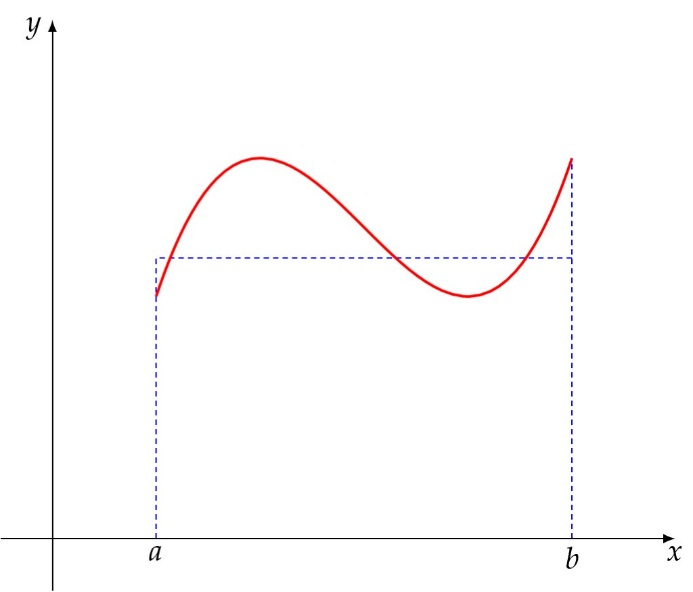1. 常数函数c于[a,b]上所有点的平均高度显然是$\lim_{n \rightarrow \infty}\frac{f(\xi_{1}) + f(\xi_{2}) + \cdots + f(\xi_{n})}{n} = c$，这与我们的直觉一致；

2. y=2x于[1,5]上的所有点的平均高度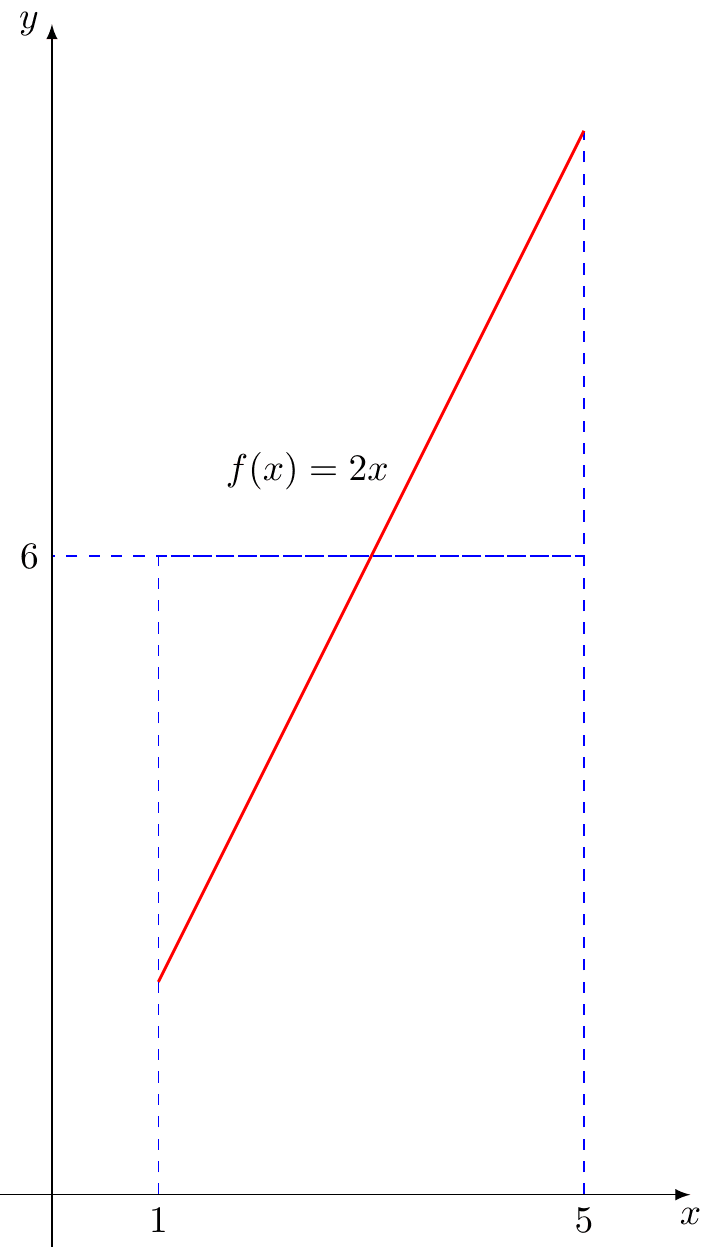从直观上来看我们极有可能认为这个平均高度是$\frac{左端点的高度 + 右端点的高度}{2} = \frac{2 \times 1 + 2 \times 5}{2} = 6$，用我们计算平均高度的方法得到这个值等于梯形的面积$\frac{(2 + 10) \cdot 4}{2} = 24$除以区间[1,5]的长度4结果是$6 = \frac{24}{4}$，也和我们的直觉一致；

1. 我们可能会从直觉上认为$\frac{1}{4}$圆的图像于第一象限内所有点的平均高度是$\frac{1}{2}$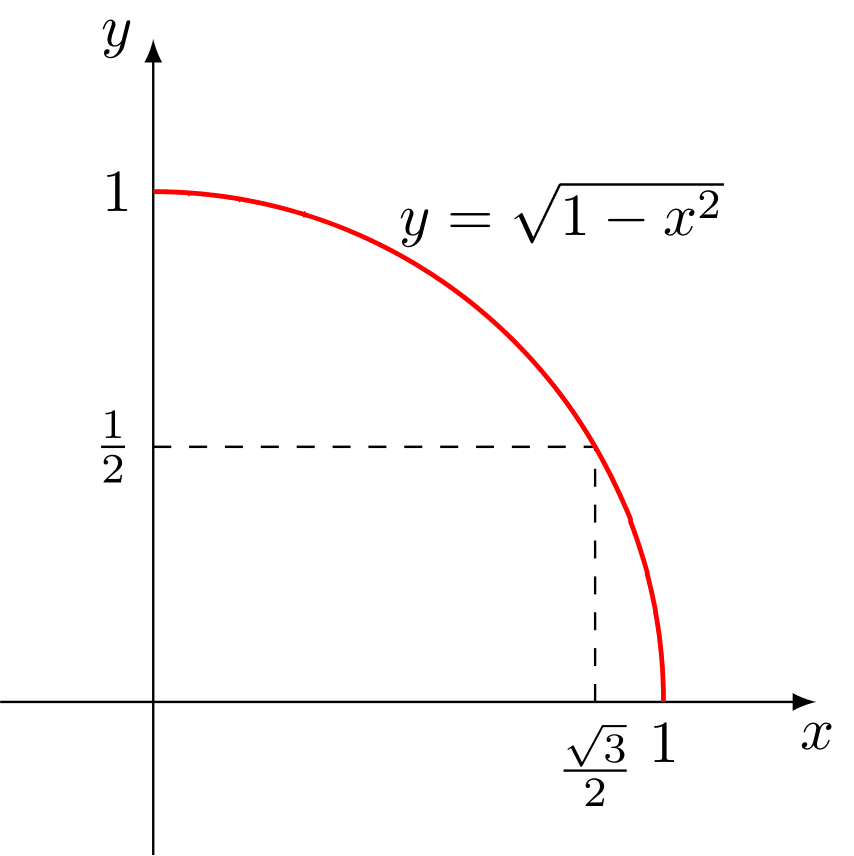但根据我们上面的方法其平均高度是$\frac{\int_{0}^{1}{\sqrt{1 - x^{2}}\text{dx}}}{1} = \int_{0}^{1}{\sqrt{1 - x^{2}}\text{dx}} = \frac{\pi}{4} \approx 0.79 > \frac{1}{2}$，那么我们的直觉错在哪里呢？$y = \sqrt{1 - x^{2}} = \frac{1}{2}$时，$x = \frac{\sqrt{3}}{2} \approx 87\%$，所以$\sqrt{1 - x^{2}}$于[0,$\ \frac{\sqrt{3}}{2}$]的图像上每个点的高度都大于等于$\frac{1}{2}$，这大约占了函数图像的87%，但我们还认为整个（100%）函数图像上所有点的高度是$\frac{1}{2}$，这就低估了这个平均高度了。

1. 由于本人不擅长数学绘图，所以本动图和情景有点不太符合，有能力者可联系我一起出更好的图

2. Introduction to Calculus and Analysis Volume I, Reprint of the 1989 edition, Richard Courant, Fritz John, p141

posted @ 2020-06-21 15:27  iMath  阅读(955)  评论(0编辑  收藏  举报